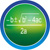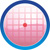6. GEOMETRY
6.1 Introduction(Definitions, basic constructions)             8
6.2 Axioms, Postulates and Enunciation on lines(Intercepts Property, Dividing a line in the given ratio) 8 8,9 8,9 8,9   8,10 9
6.3 Theorems on parallel lines 8   8,9 9   8 9
6.4 Triangles( Classification/Theorem/ Construction/ Congruency of Triangles, Base Angle Theorem, Postulates on congruent triangles) 8 9 8,9 8,9,10   8,9 8,10
6.5 Concurrent lines of triangles(Altitudes, Orthocenter, Median, Centroid, Perpendicular, Angular bisectors, Circumcenter, Incenter:Constructions) 9 10 10 9,10 10 8,9,10 8,9
6.6 Polygons(Construction of inscribed regular polygon) 9   8,9,10   9 8
6.7 Quadrilaterals(Properties, construction, Heron's formula for area of triangle, Area of a quadrilateral, Types of quadrilaterals) 8,9 8,9 8,9 8   8 8,9
6.8 Parallelogram(Construction, Area of parallelogram, Construction of Rhombus, Area of Rhombus, Construction of Trapezium, Area of Trapezium, Theorems on parallelograms, Mid point theorem) 8,9 8,9 8,9 9   8,9 8,9
6.9 Circles - Part 1(Theorems, cyclic quadrilateral, Construction of a cyclic Quadrilateral, Area of circle) 9 8,9,10 8,9,10 8,9,10 9,10 8,9,10 10
6.10 Geometric Solid Figures(Properties, Surface Area of Prisms, Pyramids, Volume of Prisms, Pyramid) 9 10     9,10 9 8
6.11 Circles - Part 2(Construction of chords, tangents) 10 10   8,10 10 10 10
6.12 Circles - Part 3(Arcs, Congruency of arcs, Length, Area of arcs)   10 10 10 9 8 8
6.13 Theorems on Triangles(Basic proportionality theorem, Theorem on equiangular , similar triangles, Postulates on similar triangles, Pythagoras Theorem,Concurrency theorems, Area of special triangles, size transformation) 10 9,10 10 8,10 9,10 8,10 10
6.14 Theorems on Circles(Touching circles, Theorem on tangents) 10 10 10 10 10 10 10
6.15 Solid figures(Cylinder, cones, spheres their surface areas, volume) 10 8,9 10 8,10 9,10 9 8,10
6.16 Scale (Surveyors) Drawing 10     8   8 9